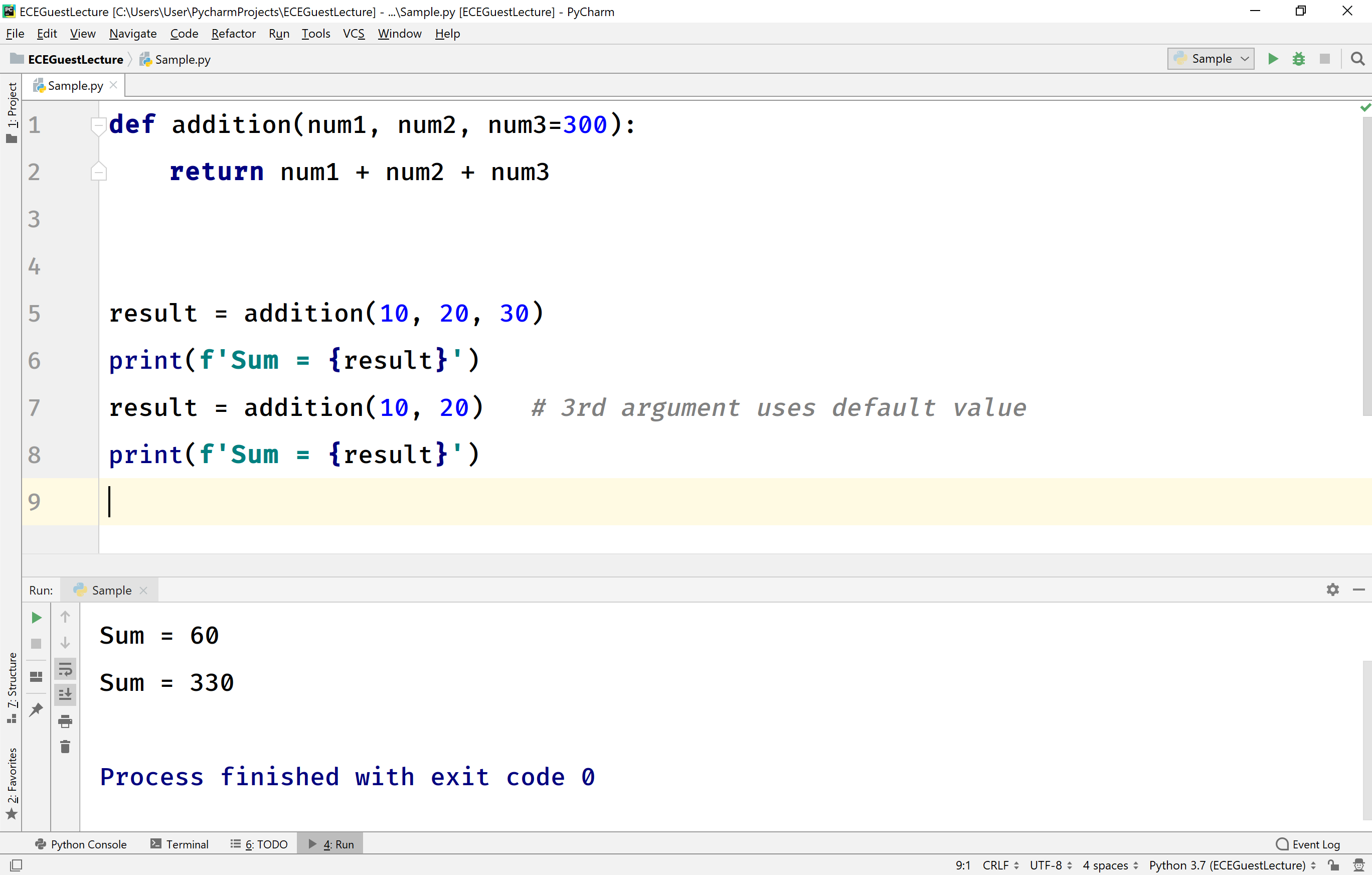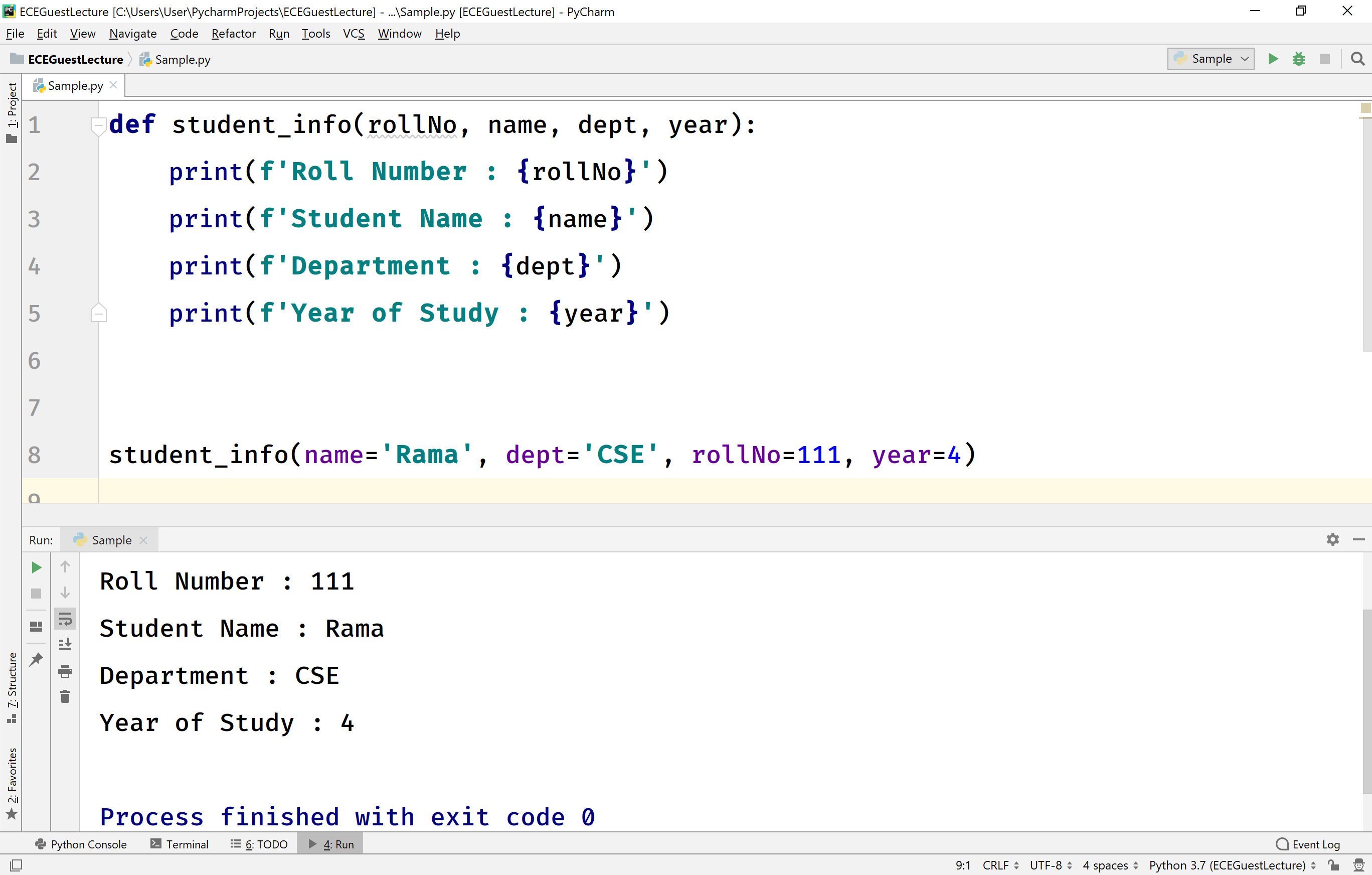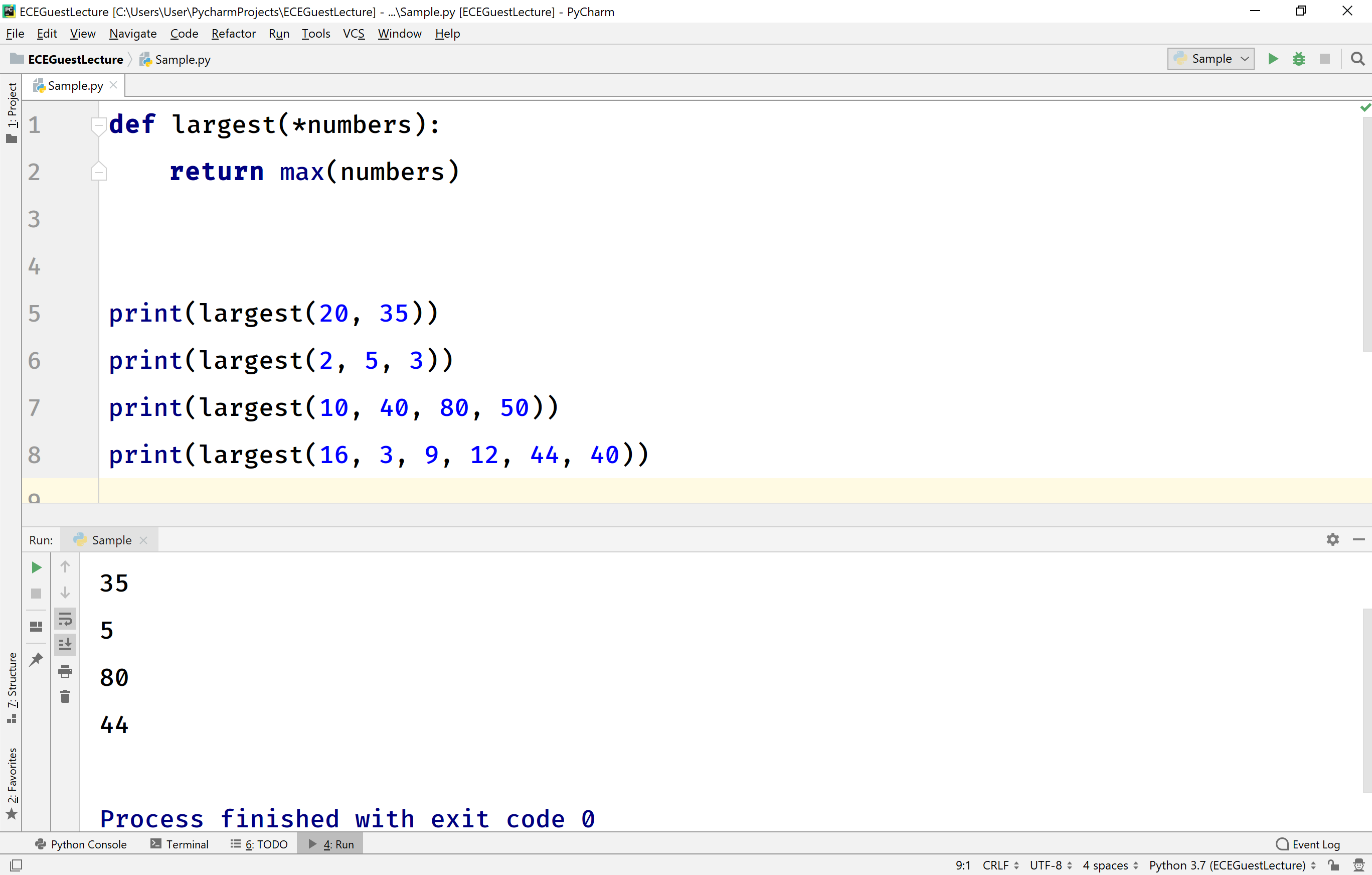The perfect place for easy learning...

×

# Python Function Arguments

In Python, there are different ways to pass arguments to a function. They are as follows.

• Positional Arguments (or) Required Arguments
• Default Arguments
• Keyword Arguments
• Variable-length Arguments

# Positional Arguments (or) Required Arguments

The positional arguments are the arguments passed to a function in the same positional order as they defined in the function definition. Here, the number of arguments and order of arguments in the function call should exactly match with the respective function definition. If any mismatch leads to error.
The positional arguments are also known as required arguments.

Consider the following example code.

# Python code to illustrate positional arguments.

``````def addition(num1, num2, num3):
return num1 + num2 + num3

result = addition(10, 20, 30)
print(f'Sum = {result}')

#result = addition(10, 20)   # Error
print(f'Sum = {result}')```
```

When we run the above example code, it produces the following output.# Default Arguments

The default argument is an argument which is set with a default value in the function definition. If the function is called with value then, the function executed with provided value, otherwise, it executed with the default value given in the function definition.

Consider the following example code.

# Python code to illustrate default arguments.

``````def addition(num1, num2, num3=300):
return num1 + num2 + num3

result = addition(10, 20, 30)
print(f'Sum = {result}')
result = addition(10, 20)   # 3rd argument uses default value
print(f'Sum = {result}')```
```

When we run the above example code, it produces the following output.# Keyword Arguments

The keyword argument is an argument passed as a value along with the parameter name (parameter_name = value). When keyword arguments are used, we may ignore the order of arguments. We may pass the arguments in any order because the Python interpreter uses the keyword provided to match with the respective parameter.

Consider the following example code.

# Python code to illustrate keyword arguments.

``````def student_info(rollNo, name, dept, year):
print(f'Roll Number : {rollNo}')
print(f'Student Name : {name}')
print(f'Department : {dept}')
print(f'Year of Study : {year}')

student_info(name='Rama', dept='CSE', rollNo=111, year=4)```
```

When we run the above example code, it produces the following output.# Variable-length Arguments

Some times we may not aware of the number of arguments to be passed to a function definition, or it may change according to the situation. The Python provides variable-length of arguments which enable us to pass an arbitrary number of arguments. Here, all the arguments are stored as a tuple of parameters in the function definition. And they are accessed using the index values (similar to a tuple).

Consider the following example code.

# Python code to illustrate keyword arguments.

``````def largest(*numbers):
return max(numbers)

print(largest(20, 35))
print(largest(2, 5, 3))
print(largest(10, 40, 80, 50))
print(largest(16, 3, 9, 12, 44, 40))```
```

When we run the above example code, it produces the following output.